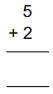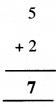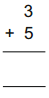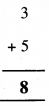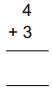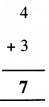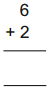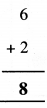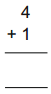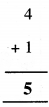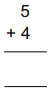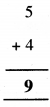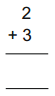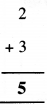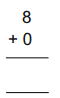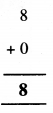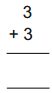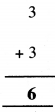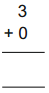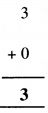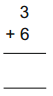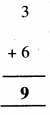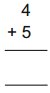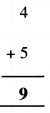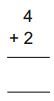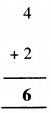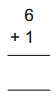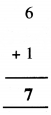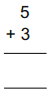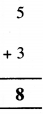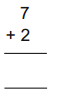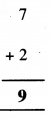# AP Board 1st Class Maths Solutions 2nd Lesson Addition

## AP State Syllabus 1st Class Maths Solutions Chapter 2 Addition

Textbook Page No. 30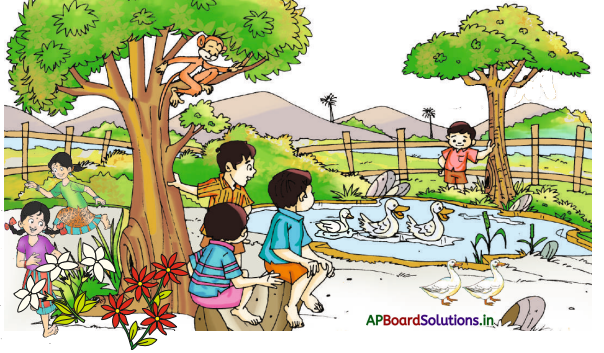Observe the above picture. Answer the following questions.

Question 1.
How many ducks are there in the pond ?
Three (3)

Question 2.
How many ducks are outside the pond ?
Two (2)Question 3.
How many ducks are there in total ?
Five (5)

3 and 2 make 5

Combining or putting different group of things together is called addition.

Question 1.
How many red flowers are there ?
Four (4)

Question 2.
How many white flowers are there ?
Three (3)

Question 3.
How many flowers are there in total ?
Seven (7)

4 and 3 make 7

Question 1.
How many girls are playing in the park ?
Two (2)

Question 2.
How many boys are playing in the park?
Four (4)

Question 3.
How many children are playing in the park in total ?
Six (6)

2 and 4 make 6

Textbook Page No. 31

Look at the first picture in each row. Add one bead to each and say the total. Write it in the third box.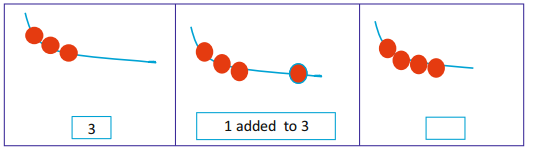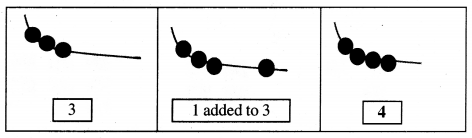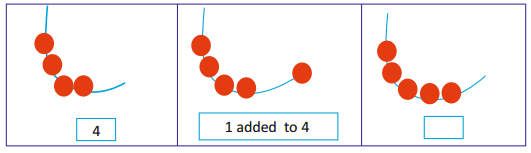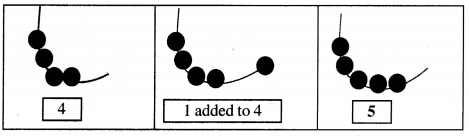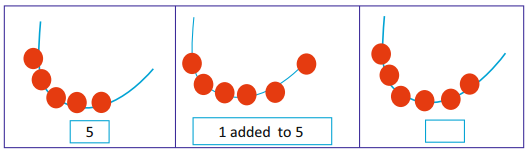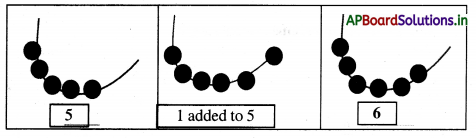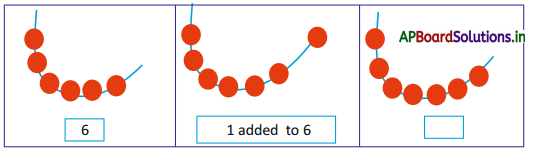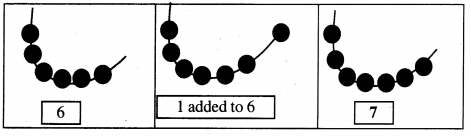Textbook Page No. 32

Count and Write. One is done for you.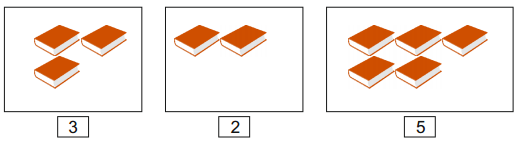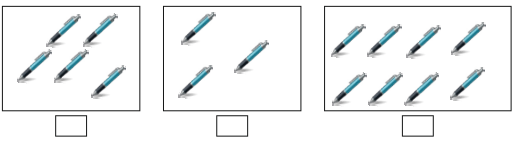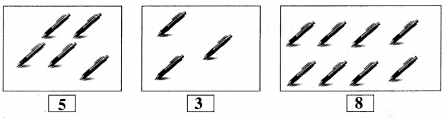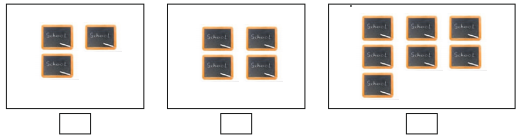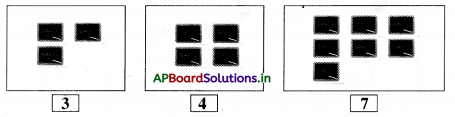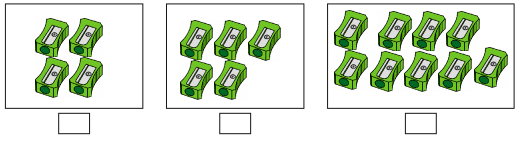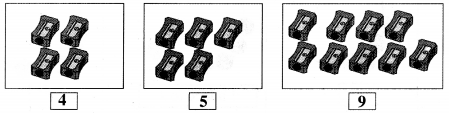4 and 5 make 9. It is written as 4 + 5 = 9

Textbook Page No. 33

Do the following sums. One example is given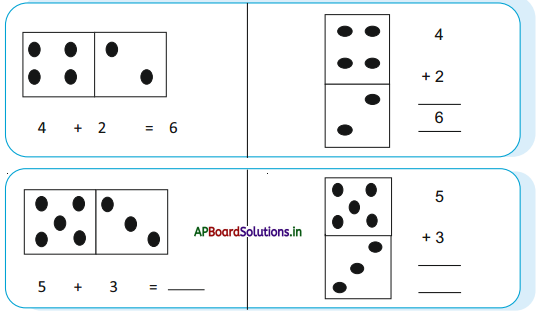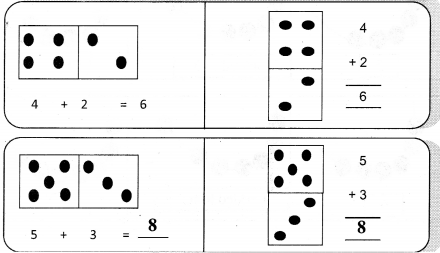Textbook Page No. 34

Count and write. One is done for you.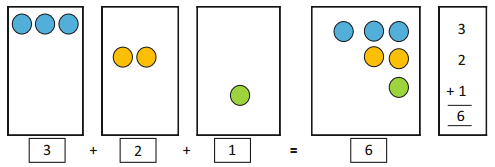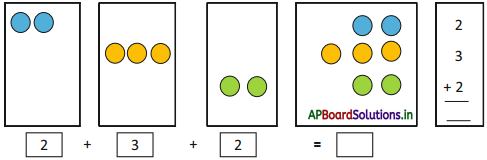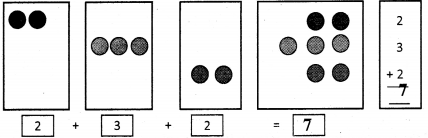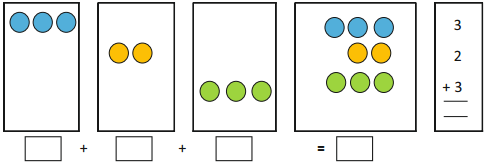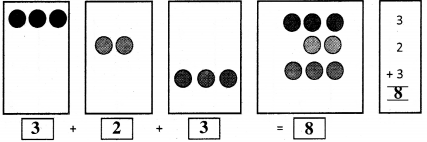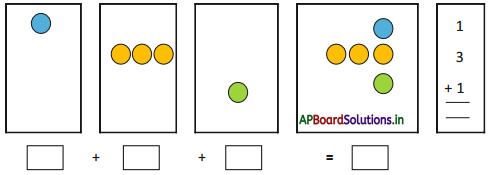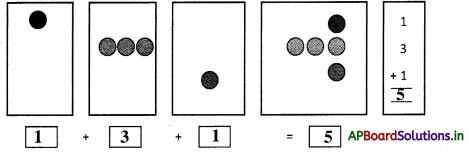Try this :

1)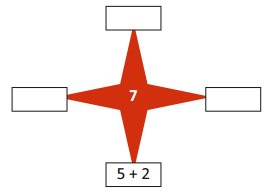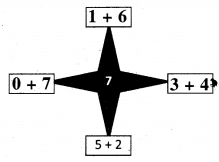2)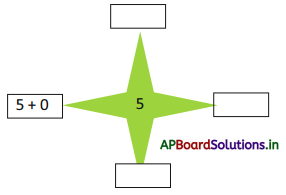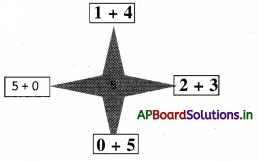Textbook Page No. 35

Exercise

Question 1.
Add the two numbers and write in the space given.
3 + 2 = 5
5 + 1 = ____
4 + 0 = ____
7 + 2 = ____
3 + 4 = ____
0 + 6 = ____
6 + 3 = ____
2 + 4 = ____
4 + 1 = ___
0 + 8 = ___
2 + 2 = ____
7 + 1 = ____

2. Add the two numbers and write in the space given.